# Search Results (7)

View
Selected filters:
• Higher Education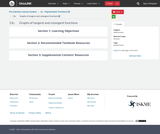Conditions of Use:
Remix and Share
Rating

Tangent and Cotangent Functions - period, phase-shift, periodic functions, asymptotes, sine and cosine functionsTMM 002 PRECALCULUS (Revised March 21, 2017)1. Functions: 1a. Analyze functions. Routine analysis includes discussion of domain, range, zeros, general function behavior (increasing, decreasing, extrema, etc.), as well as periodic characteristics such as period, frequency, phase shift, and amplitude. In addition to performing rote processes, the student can articulate reasons for choosing a particular process, recognize function families and anticipate behavior, and explain the implementation of a process (e.g., why certain real numbers are excluded from the domain of a given function).*

Subject:
Higher Education
Mathematics
Calculus
Trigonometry
Material Type:
Module
Provider:
Ohio Open Ed Collaborative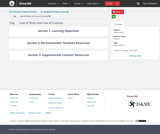Conditions of Use:
Remix and Share
Rating

Law of SinesTMM 002 PRECALCULUS (Revised March 21, 2017)2c. Analyze general triangles. Routine analysis of side lengths and angle measurements using trigonometric ratios/functions, as well as other relationships.*Sample Tasks:The student can solve general triangles using trigonometric ratios and relationships including laws of sine and cosine.The student can compare similar triangles.The student can compute length and angle measurements inside complex drawings involving multiple geometric objects.The student can algebraically describe relationships inside complex drawings involving multiple geometric objects.

Subject:
Higher Education
Mathematics
Calculus
Geometry
Trigonometry
Material Type:
Module
Provider:
Ohio Open Ed Collaborative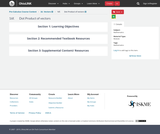Conditions of Use:
Remix and Share
Rating

Vectors - dot product, projection, decomposition of a vectorTMM 002 PRECALCULUS (Revised March 21, 2017)AdditionalOptional Learning Outcomes:2. Geometry: The successful Precalculus student can:2e. Interpret the result of vector computations geometrically and within the confines of a particular applied context (e.g., forces).Sample Tasks:The student can define vectors, their arithmetic, their representation, and interpretations.The student can decompose vectors into normal and parallel components.The student can interpret the result of a vector computation as a change in location in the plane or as the net force acting on an object.

Subject:
Engineering
Higher Education
Mathematics
Geometry
Trigonometry
Material Type:
Module
Provider:
Ohio Open Ed Collaborative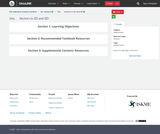Conditions of Use:
Remix and Share
Rating

Vectors - magnitude, direction, component form, trigonometric form, unit vector, algebra of vectors, applications,TMM 002 PRECALCULUS (Revised March 21, 2017)AdditionalOptional Learning Outcomes:2. Geometry: The successful Precalculus student can:2e. Interpret the result of vector computations geometrically and within the confines of a particular applied context (e.g., forces).Sample Tasks:The student can define vectors, their arithmetic, their representation, and interpretations.The student can decompose vectors into normal and parallel components.The student can interpret the result of a vector computation as a change in location in the plane or as the net force acting on an object.

Subject:
Higher Education
Mathematics
Calculus
Geometry
Physics
Material Type:
Module
Provider:
Ohio Open Ed CollaborativeConditions of Use:
Remix and Share
Rating

Ellipse - conic section, foci, major and minor axes, vertices, standard form, eccentricityTMM 002 PRECALCULUS (Revised March 21, 2017)AdditionalOptional Learning Outcomes:2. Geometry: The successful Precalculus student can:2f. Represent conic sections algebraically via equations of two variables and graphically by drawing curves.Sample Tasks:The student can perform the process “completing the square” transforming the equation into a standard form.The student can draw curves representing conic sections.The student can solve systems of equations involving linear and quadratic functions.The student can parametrize conic curves.

Subject:
Higher Education
Mathematics
Algebra
Geometry
Astronomy
Material Type:
Module
Provider:
Ohio Open Ed Collaborative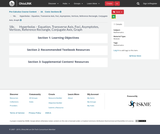Conditions of Use:
Remix and Share
Rating

Hyperbola - conic section, foci, transverse and conjugate axes, vertices, asymptotes, standard formTMM 002 PRECALCULUS (Revised March 21, 2017)AdditionalOptional Learning Outcomes:2. Geometry: The successful Precalculus student can:2f. Represent conic sections algebraically via equations of two variables and graphically by drawing curves.Sample Tasks:The student can perform the process “completing the square” transforming the equation into a standard form.The student can draw curves representing conic sections.The student can solve systems of equations involving linear and quadratic functions.The student can parametrize conic curves.

Subject:
Higher Education
Mathematics
Geometry
Astronomy
Material Type:
Module
Provider:
Ohio Open Ed CollaborativeConditions of Use:
Remix and Share
Rating

Parabola - conic section, focus, directrix, focal length, standard form, vertex form, completing the square method.TMM 002 PRECALCULUS (Revised March 21, 2017)AdditionalOptional Learning Outcomes:2. Geometry: The successful Precalculus student can:2f. Represent conic sections algebraically via equations of two variables and graphically by drawing curves.Sample Tasks:The student can perform the process “completing the square” transforming the equation into a standard form.The student can draw curves representing conic sections.The student can solve systems of equations involving linear and quadratic functions.The student can parametrize conic curves.

Subject:
Higher Education
Mathematics
Algebra
Geometry
Material Type:
Module
Provider:
Ohio Open Ed Collaborative